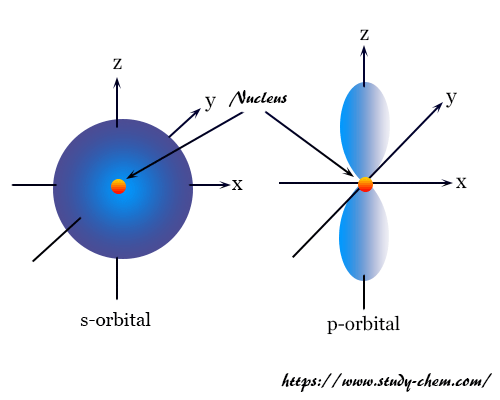Home Atom Orbital

# Orbital

Definition, Diagram, Meaning## Definition of Orbital

Contents

Orbital in chemistry or physics defines a three-dimensional region where the probability of finding an electron is maximum. The wave function suggests that the probability of finding an electron in three-dimensional space around the nucleus involves two aspects, radial probability, and angular probability.

The square of the angular part of the wave function indicates the probability of finding the electron at any distance r from the nucleus. The square of the angular part of the wave function gives the probability of finding an electron in a particular direction from the nucleus. If the radial and angular dependence of wave function is taken together, it defines the three-dimensional standing electron wave or cloud or orbital which predicts the size shape, and orientation of atomic orbitals.The pictorial representation of an orbital in two-dimensional space is difficult. It is generally represented by a shaded picture in which the intensity of shading proportional to the portability of finding the electron at that location.

### Quantum Number Orbital Diagram

In order to describe the size, shape, and orientation of orbital, three quantum number is necessary. These quantum numbers are designated by the principal (n), azimuthal (l), and magnetic (m) quantum numbers. In study chemistry, the relation between quantum number and orbital designation is represented in the below table.

 Principal quantum number (n) Azimuthal quantum number (l) Magnetic quantum number (m) n=1 (K-shell) l = 0 (1s) m = 0 (2s) n = 2 L-shell l = 0 (2s) l = 1 (2p) m = 0 (2px) m = +1 (2py) m = -1 (2pz) n =3 M-shell l = 0 (3s) m = 0 (3s) l = 1 (3p) m = 0 (3px) m = +1 (3py) m = -1 (2pz) l = 2 (3d) m = 0 (3dxy) m = +1 (3dyz) m = -1 (3dxz) m = +2 (3dx2-y2) m = -2 (3dz2)

### Shape of s-orbital

From the above table, we have seen that for s-orbitals l = 0 and m = 0. It indicates that the s-orbital has only one orientation in space with a spherically symmetrical structure. The electron cloud density in s-orbitals is not concentrated along any particular direction. Therefore, an equal chance of finding the electron density in any direction with respect to the nucleus of an atom.

### Shape of p-orbitals

For p-orbital l = 1 and m = +1, 0, -1. Three values of magnetic quantum number (m) define the three orientations along x, y, z-direction in space. Therefore, p-orbitals are designated as px, py, and pz. In the absence of a magnetic or electric field, these three orbitals are equivalent in energy and said to three-fold degenerate or triply degenerate energy level.

The three p-orbitals are dumb-bell shape which is perpendicular to each other. Each of the three p-orbitals has two lobes touching each other at the origin and these lobes are completely symmetrical along any of the three-axis. For example, the px-orbital is symmetrical to the x-axis. The plane which separated the two lobes of the p-orbital is called the nodal plane. The electron density on the nodal plane is zero. For px-orbital, yz-plane is the nodal plane.

### Shape of d-orbitalsd-orbital arise when n = 3 and m = +2, +1, 0, -2, -1 or it starts with the 3rd main energy level. The azimuthal quantum number indicating that d-orbitals have five orientations in space. These five orientations along the x, y, z-axis named dxy, dxz, dyz, dx2-y2, and dz2. The three orbitals namely dxy, dyz, dxz have their lobes lying symmetrically between their respective coordinate axis. For example, the lobes of the dxy orbital lying between the x and y-axis. This set of three orbitals is known as the t2g set non-axial set.

The lobes of dx2-y2 are lying along the x and y-axis and the lobes of dz2 are lying along the z-axis. This set of two orbitals are called eg set or axial set.